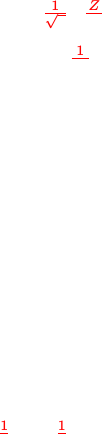Study Guides (400,000)
US (230,000)
UC-Irvine (3,000)
CHEM (100)
Quiz

# CHEM 132B Study Guide - Quiz Guide: Spherical Harmonics, Term Symbol, Haplogroup R2AExam

Department
Chemistry
Course Code
CHEM 132B
Professor
Eric Potma
Study Guide
Quiz

This preview shows page 1. to view the full 5 pages of the document.
Chem 132B Practice Quiz 2 Key
Version B
Problem 1
Encircle all that are true regarding the spherical harmonic wavefunctions.
a) The energy associated with the spherical harmonic wavefunctions is inde-
pendent of the quantum number ml.True
b) The magnitude of orbital angular momentum is not quantized, but its
projection onto a chosen axis is. False
c) If we determine the angular momentum of the spherical harmonic wave-
function along x, then its projections along zand yare unknown. True
d) We cannot both determine the magnitude of orbital angular momentum
and its projection onto the z-axis at the same time. False
Problem 2
Encircle all that are true.
a) The groundstate of hydrogen is 2S0.False
b) The groundstate of helium is 1S0.True
c) The groundstate of He+is 2S1/2.True
d) The groundstate of His 2P1/2.False
1

Unlock to view full version

Only page 1 are available for preview. Some parts have been intentionally blurred.Problem 3
An excited hydrogen atom is the 2s1conﬁguration.
a) Give an expression for
1) the radial part of the wavefunction (Rn,l(r)).
2) the angular part of the wavefunction (Yl,ml(θ, φ)).
R2,0(r) = 1
8Z
a03/2(2 r/a0)er/2a0
Y0,0(θ, φ) = 1
4π1/2
b) Determine the location (r > 0) along rwhere there is zero probability of
ﬁnding the electron (in units of a0).
Radial node is found when R2,0(r) = 0, which is when (2 r/a0) = 0.
This gives r= 2a0
c) Give the term symbol of the 2s1conﬁguration.
l= 0, s =1
2, j =1
2, which gives 2S1/2
d) Can the atom decay to the ground state through the emission of a photon?
No, ∆l6=±1.
2